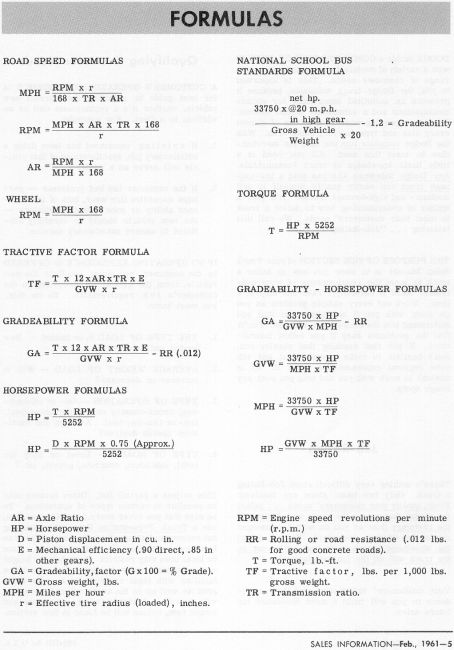The numbers don't lie - by Clint "Junior" Dixon

Folks are always asking how fast they can drive a Power-Wagon fitted with a certain diameter of tire, or with 4.89:1 gearing verses 5.83:1 gearing, or with a V-8 engine in place of the stock L-head 6.

Here is a list of formulas from which one can figure an unknown by plugging several knowns into the proper formula. Keep in mind that overall tire diameter is basically meaningless in these examples. What you have to know is the effective tire radius in inches with the truck normally loaded. This is sometimes referred to as "rolling radius" and is represented by the placeholder “r” in the formulas. This can be measured from level hard concrete to the center of a rear axle flange, tires inflated correctly and truck loaded normally.

One can also figure a close approximation of the tire size that will be required when they know what speed they are planning to drive, at what engine rpm they wish to accomplish this, and the gearing they are planning to use. This formula is not included in the list, but goes like this:

Take the desired mph that you wish to travel at and multiply it by the axle ratio you plan to be using. Then multiply this product by the transmission ratio you plan to select (normally “1” if you are using a 4-speed transmission with direct drive 4th gear, and you are calculating your answer based on actually driving while in 4th gear). Then multiply this product by 168. You will now have an end product into which you will divide the rpm that you plan to have the engine turning at (usually the most efficient operating speed of the engine). The remainder is the effective tire radius that would be required under the conditions plugged into the formula above. You can multiply this “rolling radius” by 2 to get a close approximation of the tire diameter required to meet all of the specified variables in the formula above.

Because these formulas take into account both the truck’s axle ratio and transmission ratios, one can arrive at accurate answers even when using 2-speed rear axles, overdrive transmissions, and even with a transmission in any available gear, including reverse, if one has a particular need for that information. One can even take into account the reduction of a 2-speed transfer case and arrive at accurate answers by plugging that information into the formula if desired. Just remember that any component located between the flywheel and tire that can potentially change rotational speed must be plugged into the desired formula. I.E.: if you desire an answer based on the fact that you will be driving your truck in reverse gear, while your overdrive box is in “high range”, your transfer case is in “low range”, and your 2-speed differential is shifted into “high range”, then you must plug all of this information into the multiplying part of the formulas that read “---- x AR x TR x 168”. In this example, you would have to know the gear reduction of reverse (probably around 6.0:1 or “6”), overdrive ratio (maybe around 1:1.20 or “.83”), transfer case ratio (probably around 1.96:1 or “1.96”), rear differential (maybe around 4.375:1 “4.38”). Just keep multiplying together and then multiply the product by 168.

Junior